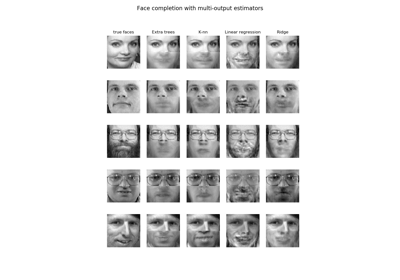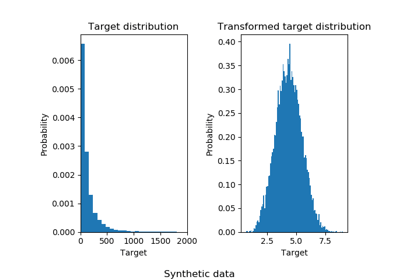/scikit-learn

# 3.2.4.1.9. sklearn.linear_model.RidgeCV

`class sklearn.linear_model.RidgeCV(alphas=(0.1, 1.0, 10.0), fit_intercept=True, normalize=False, scoring=None, cv=None, gcv_mode=None, store_cv_values=False)` [source]

Ridge regression with built-in cross-validation.

By default, it performs Generalized Cross-Validation, which is a form of efficient Leave-One-Out cross-validation.

Read more in the User Guide.

Parameters: `alphas : numpy array of shape [n_alphas]` Array of alpha values to try. Regularization strength; must be a positive float. Regularization improves the conditioning of the problem and reduces the variance of the estimates. Larger values specify stronger regularization. Alpha corresponds to `C^-1` in other linear models such as LogisticRegression or LinearSVC. `fit_intercept : boolean` Whether to calculate the intercept for this model. If set to false, no intercept will be used in calculations (e.g. data is expected to be already centered). `normalize : boolean, optional, default False` This parameter is ignored when `fit_intercept` is set to False. If True, the regressors X will be normalized before regression by subtracting the mean and dividing by the l2-norm. If you wish to standardize, please use `sklearn.preprocessing.StandardScaler` before calling `fit` on an estimator with `normalize=False`. `scoring : string, callable or None, optional, default: None` A string (see model evaluation documentation) or a scorer callable object / function with signature `scorer(estimator, X, y)`. `cv : int, cross-validation generator or an iterable, optional` Determines the cross-validation splitting strategy. Possible inputs for cv are: None, to use the efficient Leave-One-Out cross-validation integer, to specify the number of folds. An object to be used as a cross-validation generator. An iterable yielding train/test splits. For integer/None inputs, if `y` is binary or multiclass, `sklearn.model_selection.StratifiedKFold` is used, else, `sklearn.model_selection.KFold` is used. Refer User Guide for the various cross-validation strategies that can be used here. `gcv_mode : {None, ‘auto’, ‘svd’, eigen’}, optional` Flag indicating which strategy to use when performing Generalized Cross-Validation. Options are: ```'auto' : use svd if n_samples > n_features or when X is a sparse matrix, otherwise use eigen 'svd' : force computation via singular value decomposition of X (does not work for sparse matrices) 'eigen' : force computation via eigendecomposition of X^T X ``` The ‘auto’ mode is the default and is intended to pick the cheaper option of the two depending upon the shape and format of the training data. `store_cv_values : boolean, default=False` Flag indicating if the cross-validation values corresponding to each alpha should be stored in the `cv_values_` attribute (see below). This flag is only compatible with `cv=None` (i.e. using Generalized Cross-Validation). `cv_values_ : array, shape = [n_samples, n_alphas] or shape = [n_samples, n_targets, n_alphas], optional` Cross-validation values for each alpha (if `store_cv_values=True` and `cv=None`). After `fit()` has been called, this attribute will contain the mean squared errors (by default) or the values of the `{loss,score}_func` function (if provided in the constructor). `coef_ : array, shape = [n_features] or [n_targets, n_features]` Weight vector(s). `intercept_ : float | array, shape = (n_targets,)` Independent term in decision function. Set to 0.0 if `fit_intercept = False`. `alpha_ : float` Estimated regularization parameter.

`Ridge`
Ridge regression
`RidgeClassifier`
Ridge classifier
`RidgeClassifierCV`
Ridge classifier with built-in cross validation

#### Examples

```>>> from sklearn.datasets import load_diabetes
>>> from sklearn.linear_model import RidgeCV
>>> clf = RidgeCV(alphas=[1e-3, 1e-2, 1e-1, 1]).fit(X, y)
>>> clf.score(X, y)
0.5166...
```

#### Methods

 `fit`(X, y[, sample_weight]) Fit Ridge regression model `get_params`([deep]) Get parameters for this estimator. `predict`(X) Predict using the linear model `score`(X, y[, sample_weight]) Returns the coefficient of determination R^2 of the prediction. `set_params`(**params) Set the parameters of this estimator.
`__init__(alphas=(0.1, 1.0, 10.0), fit_intercept=True, normalize=False, scoring=None, cv=None, gcv_mode=None, store_cv_values=False)` [source]
`fit(X, y, sample_weight=None)` [source]

Fit Ridge regression model

Parameters: `X : array-like, shape = [n_samples, n_features]` Training data `y : array-like, shape = [n_samples] or [n_samples, n_targets]` Target values. Will be cast to X’s dtype if necessary `sample_weight : float or array-like of shape [n_samples]` Sample weight `self : object`
`get_params(deep=True)` [source]

Get parameters for this estimator.

Parameters: `deep : boolean, optional` If True, will return the parameters for this estimator and contained subobjects that are estimators. `params : mapping of string to any` Parameter names mapped to their values.
`predict(X)` [source]

Predict using the linear model

Parameters: `X : array_like or sparse matrix, shape (n_samples, n_features)` Samples. `C : array, shape (n_samples,)` Returns predicted values.
`score(X, y, sample_weight=None)` [source]

Returns the coefficient of determination R^2 of the prediction.

The coefficient R^2 is defined as (1 - u/v), where u is the residual sum of squares ((y_true - y_pred) ** 2).sum() and v is the total sum of squares ((y_true - y_true.mean()) ** 2).sum(). The best possible score is 1.0 and it can be negative (because the model can be arbitrarily worse). A constant model that always predicts the expected value of y, disregarding the input features, would get a R^2 score of 0.0.

Parameters: `X : array-like, shape = (n_samples, n_features)` Test samples. For some estimators this may be a precomputed kernel matrix instead, shape = (n_samples, n_samples_fitted], where n_samples_fitted is the number of samples used in the fitting for the estimator. `y : array-like, shape = (n_samples) or (n_samples, n_outputs)` True values for X. `sample_weight : array-like, shape = [n_samples], optional` Sample weights. `score : float` R^2 of self.predict(X) wrt. y.
`set_params(**params)` [source]

Set the parameters of this estimator.

The method works on simple estimators as well as on nested objects (such as pipelines). The latter have parameters of the form `<component>__<parameter>` so that it’s possible to update each component of a nested object.

Returns: self

## 3.2.4.1.9.1. Examples using `sklearn.linear_model.RidgeCV`Face completion with a multi-output estimatorsEffect of transforming the targets in regression model